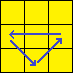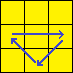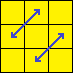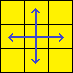# 3×3 PLL Algorithms PDF

4 People Like This
REPORT THIS PDF ⚐

## 3×3 PLL Algorithms PDF

 Algorithm Name Image Algorithm Ua perm[R U’ R] U R U R U’ R’ U’ R2 (y2) R2 U’ R’ U’ R U R U R U’ R Ub permR2 U R U R’ U’ R’ U’ R’ U R’ (y2) R’ U R’ U’ R’ U’ R’ U R U R2 Z permM2 U M2 U M’ U2 M2 U2 M’ U2 (y) [U R’ U’] R U’ R U R U’ R’ U R U [R2 U’ R’ U] H permM2 U M2 U2 M2 U M2